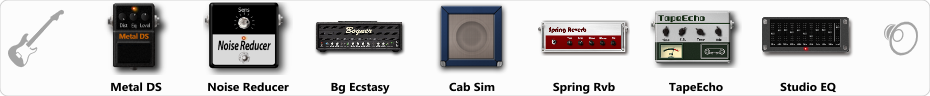# Ritm-Solo

Discussion in 'ToneLib-GFX presets' started by SergMus, Dec 11, 2022.

1. Ritm-Solo

Effects chain:Effect: "Metal DS" (Overdrive / Distortion), active - "yes"
"Dist" = 82
"Bass" = 74
"Middle" = 59
"Treble" = 55
"Level" = 57

Effect: "Noise Reducer" (Dynamics / Filter), active - "yes"
"Sens" = 92
"Mode" = Soft

Effect: "Bg Ecstasy" (Amp simulators), active - "yes"
"Gain" = 87
"Bass" = 50
"Middle" = 69
"Treble" = 60
"Presence" = 47
"Master" = 100
"Output" = 54
"Level (dB)" = 0

Effect: "Cab Sim" (Cabinets), active - "yes"
"Model" = 2x12" Silver Bell
"Level (dB)" = 0

Effect: "Spring Rvb" (Reverb), active - "yes"
"Time" = 3.1
"PreDelay" = 22
"LoDamp" = 43
"HiDamp" = 26
"Mix" = 40

Effect: "TapeEcho" (Delay), active - "yes"
"Time" = 499
"Feedback" = 38
"Tone" = 41
"LoDamp" = 41
"Mix" = 48

Effect: "Studio EQ" (Dynamics / Filter), active - "yes"
"31 Hz" = -1
"62 Hz" = 3
"125 Hz" = 3
"250 Hz" = -6
"500 Hz" = 2
"1 kHz" = -2
"2 kHz" = 2
"4 kHz" = 4
"8 kHz" = -1
"16 kHz" = 0
"above 16 kHz" = 0
"Level (dB)" = 0

Note: You will need to download and install the ToneLib-GFX software to use the preset.

File size:
622 bytes
Views:
3,347